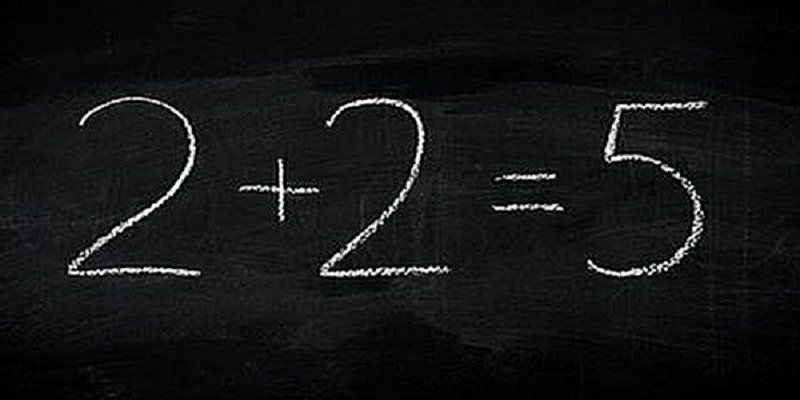Register for Maths, Science, English, Reasoning Olympiad Exams Register here | Book Free Demo for Live Olympiad Classes here | Check Olympiad Exam Dates here | Buy Practice Papers for IMO, IOM, HEO, IOEL etc here | Login here to participate in all India free mock test on every Saturday

# Amazing Mathematics Tricks That You Should Know1) Fast Multiplication by 4

To multiply any number times 4, at lightning speeds:

• First, double the number and then double it again.
• Let's use this shortcut with 1,223 times 4: double 1,223 is 2,446, and double 2,446 is 4,892.

2) Balanced Average Approach

Instead of using the average formula, you can use the balanced average approach. Think of an average as a target that all items in a list are aiming for and you are trying to balance them out to match that target. For example, let's say that you have 5 exams in your history class and you want to get at least ‘92 out of 100’. Here are your grades so far:

• First exam = 81
• Second exam = 98
• Third exam = 90
• Fourth exam = 93

What grade would you need to get on the fifth exam to get 92 as an average? Let's add up, how much you exceeded or missed your target on every attempt: - 11 + 6 - 2 + 1 equals - 6. To balance your average you need to make up for those - 6 points by making +6 points on top of your target. You need to make 98 on your fifth exam to reach your target grade of 92. Better start studying!

3) Ballpark Fractions

Estimate fractions faster by using easy benchmarks, such as ¼, ?, ½, and ¾. For example, 30⁄50 is close to 30⁄60. Since 30⁄60 is ½ and has a bigger denominator than 30⁄50, 30⁄50 must be a little bit bigger than 0.50. (The actual value is 0.60.)

4) The Always-3 Trick

Now, here is a party trick in which you can pretend to be Will Hunting.

• Ask somebody to pick a number.
• Tell them to double that number.